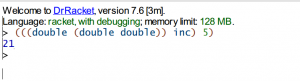# 对于SICP中1.41习题的思考

DBQ，我学着学着ObjC又去摸鱼看起了SICP（逃）

（阅读以下内容或许需要懂得Lisp的基本语法）

Lisp的语法其实很简单，就是由很多括号组成的。

foo 就是函数名，a b c 是参数名。整一个括号会变成该函数的返回值。

1.41 请定义一个过程 double，它以一个有一个参数的过程作为参数，double 返回一个过程。这一过程将原来那个参数过程应用两次。例如，若 inc 是个给参数加一的过程，(double inc) 将给参数加2.下面表达式返回什么值：

`(((double (double double)) inc) 5)`

### 为什么会这样？

``````(define (double f)
(lambda (x)
(f (f x))))``````（其实函数嵌套操作本身就是遵守结合律的，如果有更多层的话还能先求其中一小部分再结合起来一起求，不够在这里说就是题外话了）

``((double (double (double inc))) 5)``

``(((double (double double)) inc) 5)``

[(d (d (d )))(inc)](5)

inc 过程并不是最内部的过程，不能够像刚才那样分析。

``````((double f) x) 等价于 (f (f x))

((double d4) x) 等价于 (d4 (d4 x))``````

……当我写到这里的时候，我才突然知道引起这个错误的根本原因是什么。## 《对于SICP中1.41习题的思考》有4个想法

1.5:59pm说道：

如果只是误把 ((double f) x) 和 (* 2 (f x)) 等价了话，好像也得不到 13 的结果🤔？
关键就是在于 ((d (d d)) f) 不等于 (d (d (d f))) 而是等于 (d (d (d (d f)))) 吧。BTW 王垠之前发了一道练习题就是这道题 🤣

1.陆初说道：

不愧是jp大佬，一眼就看出问题真正的本质！
从 ((d (d d)) f) 展开成 (d (d (d (d f)))) 的过程能不能写一下，实在想不出来orz

1.Kawaii ... !说道：

(d d) 就两个 d 了嘛，前面再加个 d 就四个 d 了嘛（
用 S 表达式比较难描述，你改成用一般的表达式去理解可能会好一点。

2.6：00PM说道：

老师太会了！